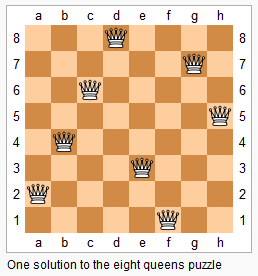# 51. N-Queens (Hard)

https://leetcode.com/problems/n-queens/

The n-queens puzzle is the problem of placing n queens on an n×n chessboard such that no two queens attack each other.Given an integer n, return all distinct solutions to the n-queens puzzle.

Each solution contains a distinct board configuration of the n-queens' placement, where `'Q'` and `'.'` both indicate a queen and an empty space respectively.

Example:

```Input: 4
Output: [
[".Q..",  // Solution 1
"...Q",
"Q...",
"..Q."],

["..Q.",  // Solution 2
"Q...",
"...Q",
".Q.."]
]
Explanation: There exist two distinct solutions to the 4-queens puzzle as shown above.
```

## Solutions

``````class Solution {

private Map<String, Boolean> validityMap = new HashMap<>();
private List<List<String>> ans = new ArrayList<>();

public List<List<String>> solveNQueens(int n) {
if (n < 1) {
return ans;
}

int [] queens = new int[n];

recurse(queens, 0);

return ans;
}

private void recurse(int [] queens, int col) {
if (col == queens.length) {
List<String> result = convert(queens);

return;
}

for (int row = 0; row < queens.length; ++row) {
for (int j = col + 1; j < queens.length; j++) {
queens[j] = -1;
}

queens[col] = row;

String key = Arrays.toString(queens);

// We use validityMap to keep the track of valid and invalid sequence so that
// we are able to reduce the computation overhead dramatically.
if (!validityMap.containsKey(key)) {
validityMap.put(key, isValid(queens, col, row));
}

// valid sequence, keep on digging into it.
if (validityMap.get(key)) {
recurse(queens, col + 1);
}
}
}

private boolean isValid(int [] queens, int col, int row) {

// col is the last index, not the length

for (int i = 0; i < col; ++i) {
// previous point (i, queens[i])

// horizontal comparision, check out if any pieces situated on same row
if (queens[i] == row) {
return false;
}

// Assume point A(a1, a2) and B(b1, b2) are diagonally arranged, abs(a1 - b1) == abs(a2 - b2).
// We can imagine that with point A and B as the diagonal points, we can form a square.
// As a square, all the edges should be of same length.

// diagonal comparision
if (Math.abs(col - i) == Math.abs(row - queens[i])) {
return false;
}
}

return true;
}

private List<String> convert(int [] queens) {
int len = queens.length;

List<String> ans = new ArrayList<>();
for (int c = 0; c < len; c++) {
String row = "";
for (int r = 0; r < len; r++) {
if (r == queens[c]) {
row += "Q";
} else {
row += ".";
}
}

}

return ans;
}
}
``````